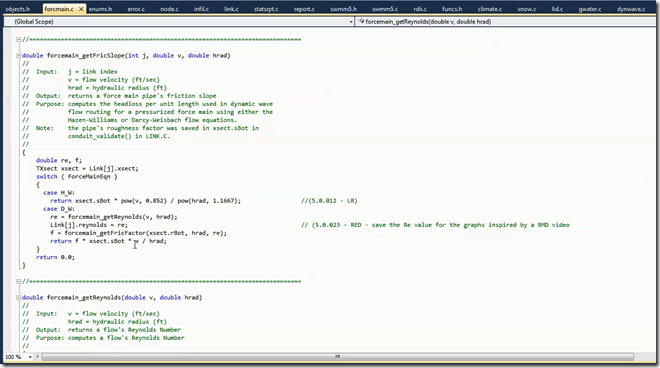Force Mains

# What are typical Reynolds Numbers in SWMM5 or InfoSWMM

The Reynolds Number is not shown in SWMM 5 of  InfoSWMM but can easily be added to the SWMM 5 code as shown in Figure 1.  The equation for the Reynolds Number isNormally, as shown is Figure 3 the Reynolds number is is very high and is indicative of very turbulent flow based on typical flows, velocities and pipe diameters/hydraulic radius's in SWMM 5 (Figure 2).Figure 1.  Reynolds Number is calculated in SWMM 5 for Darcy-Weisbach Force Main Friction Loss.Figure 2.  Reynolds Number is calculated in SWMM 5 for Darcy-Weisbach Force Main Friction Loss. Where is is the DW value entered for a Force Main.Figure 3.  Reynolds Number is calculated in SWMM 5 for Darcy-Weisbach Force Main Friction Loss, Typical values of the Reynolds Number.

Categories: Force Mains, InfoSWMM, SWMM5